vint## vint

بازدید : 713 جمعه 12 دي 1393

## vint

`(vint(psexpr,expr,top`

This function performs a mass-weighted vertical integral in mb pressure coordinates. The three arguments to `vint` are:

`psexpr   `a grads expression for the surface pressure, in mb, which bounds the integral on the bottom.
``` expr     a grads expression representing the quantity to be integrated. ``` ``` top      the bounding top pressure, in mb. This value must be a constant and cannot be provided as an expression. ```

The calculation is a sum of the mass-weighted layers:

`f/g * sum(expr * Delta(level))`

The bounds of the integration are the surface pressure and the indicated `top` value. The scale factors are f=100 and g=9.8. The summation is done for each layer present that is between the bounds. The layers are determined by the Z levels of the default file. Each layer is considered to be from the midpoints between the levels actually present, and is assumed to have the same value throughout the layer, namely the value of the gridpoint at the middle of the layer.

### Usage Notes

1. The summation is done using the Z levels from the default file, so it is important that the default file have the same Z dimension coordinates as `expr`.
2. Data levels below and above the bounds of the summation are ignored.
3. The Z dimension in world-coordinate units is assumed to be pressure values given in millibars (mb). The units of g are such that when the expression integrated is specific humidity (q) in units of g/g, the result is kg of water per square meter, or precipitable water in mm.
4. It is usually a good idea to make the `top` pressure value to be at the top of a layer, which is midway between grid points. For example, if the default file (and the data) have pressure levels of ...,500,400,300,250,... then a good value for `top` might be 275, the value at the top of the layer that extends from 350 to 275 mb.
5. The `vint` function operates only in an X-Y varying dimension environment.
6. Be sure the units of the surface pressure (argument 1) are in millibars (mb).

### Examples

1. This expression will integrate specific humidity to obtain precipitable water, in mm:

`vint(ps,q,275)`

2. This is an artificial example that demonstrates a vertical integration from a fixed lower bound of 1000mb to the top of the atmosphere, and integrating a field of all 1's. This gives an answer of 10204.1 (or 100000/9.8) which is the mass of air (in kg) of a 1 meter squared column when the surface pressure is 1 bar and the accelleration due to gravity is assumed to be exactly 9.8m/sec**2 over the entire column.

`vint(const(ps,1000),const(t,1),0)`
مطالب مرتبط
ارسال نظر برای این مطلب

نام
ایمیل (منتشر نمی‌شود) (لازم)
وبسایت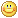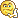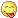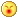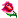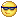نظر خصوصی
مشخصات شما ذخیره شود ؟ [حذف مشخصات] [شکلک ها]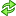کد امنیتی
اطلاعات کاربری
 نام کاربری : رمز عبور :
• فراموشی رمز عبور؟
• لینک دوستان
آرشیو
آمار سایت
• کل مطالب : 155
• کل نظرات : 0
• افراد آنلاین : 1
• تعداد اعضا : 4
• آی پی امروز : 7
• آی پی دیروز : 14
• بازدید امروز : 23
• باردید دیروز : 66
• گوگل امروز : 0
• گوگل دیروز : 0
• بازدید هفته : 272
• بازدید ماه : 2,458
• بازدید سال : 34,296
• بازدید کلی : 155,348
• کدهای اختصاصی